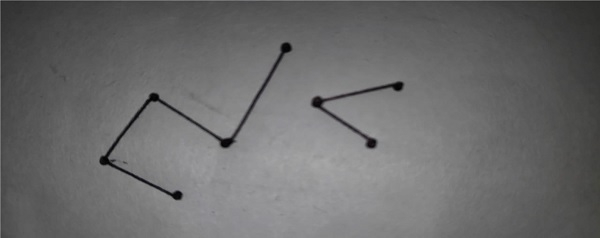# BFS for Disconnected Graph in C++

Disconnected graph is a Graph in which one or more nodes are not the endpoints of the graph i.e. they are not connected.A disconnected graph…

Now, the Simple BFS is applicable only when the graph is connected i.e. all vertices of the graph are accessible from one node of the graph. in the above disconnected graph technique is not possible as a few laws are not accessible so the following changed program would be better for performing breadth first search in a disconnected graph.

## Example

#include<bits/stdc++.h>
using namespace std;
void insertnode(vector<int> adj[], int u, int v) {
}
void breathFirstSearch(int u, vector<int> adj[], vector<bool> &visited) {
list<int> q;
visited[u] = true;
q.push_back(u);
while(!q.empty()) {
u = q.front();
cout << u << " ";
q.pop_front();
for (int i = 0; i != adj[u].size(); ++i) {
}
}
}
}
void BFSdisc(vector<int> adj[], int V) {
vector<bool> visited(V, false);
for (int u=0; u<V; u++)
if (visited[u] == false)
}
int main() {
int V = 5;
}
0 4 1 2 3